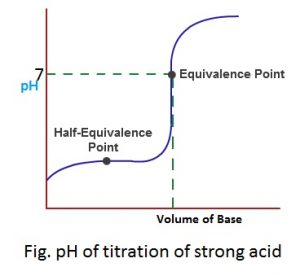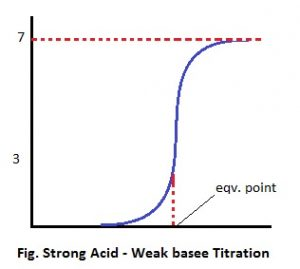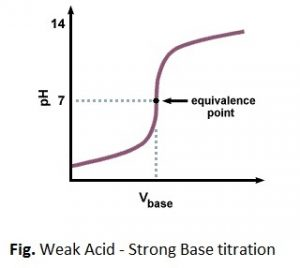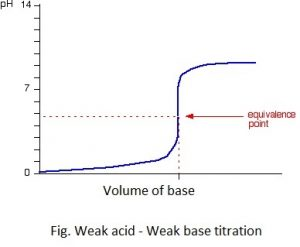# Selection Of pH Indicator (Choice Of pH Indicator)

Each pH indicator has its own pH range for its color change. It means some indicators change their color in acidic rain (pH < 7) and some change in basic rain (pH > 7)
Eg. Methylorange change its color at the pH range 3-4.5 and phenolphthalein change its color at the pH range 8-10.
On the other hand pH at the equivalent point of acid-base react may not be 7 always. In some case it is greater than 7, less than 7 or in same case it is equal to 7 and it is decided by the nature of acid and base present in the reaction.
In order to determine the accurate end point of acid-base titration, the pH indicator should be selected in such a way that the pH range for the color change of the indicator must coincide with the pH at the equivalent point of reaction.
For this consider the acid-base indicators are selected by classifying different acid-base reaction of the titration in different groups as follows:

1. Strong acid titration
Eg. HCl + NaOH → NaCl + H2O

The pH at this type of reaction is 7 (neutral) because the salt doesn’t undergo hydrolysis with water. The pH change at the end of this type of titration is 3-10 approx.

The variation of pH in course of titration with respect to the volume of base added from.

When plotted graphically, pH-titration curve is observed or obtained and the nature of pH titration curve is given below:Because of very sharp and wide pH change, any indicator either methyl orange (3-4.5) or phenolphthalein (8-10) can be used to find the actual end point of this type of titration.

2. Strong acid-weak base titration
HCl + NH4OH ⇋ NH4Cl + H2O

The pH at the equivalent point of this type of reaction is <7 (acidic) because the salt undergoes hydrolysis to give strong acid and weak base. The pH change at the end point of this type of titration is 3-7 approx. The pH titration curve is given as:The indicator used to find the end point of titration should keep the color change in acidic range. For this purpose 3-4.5 is proper indicator but not phenolphthalein is proper.

3. Weak acid-strong base titration
eg. CH3COOH + NaOH ⇋ CH3COONa + H2O

The pH at the equivalent point of this type of reaction is >7 (basic) because the salt undergoes hydrolysis to give Strong base and weak acid. The pH change at the end point of this type of titration is 7-10 approx. The pH titration curve is given as:The indicator used to find the end point of this type of titration should give the color change in basic range. For this purpose phenolphthalein is proper indicator but methylorange (3-4.5) is improper to find out the accurate end point of titration.

4. Weak acid-weak base titration
eg. CH3COOH + NH4OH ⇋ CH3COONa + H2O

The pH at the equivalent point of this type of reaction may be either slightly >7, <7 or =7 and it is decided by the relative extent of ionization of Weak Acid and weak base produced after the hydrolysis of the salt.

The pH change at the end point of this type of titration is not sharp and wide because the salt acts as a buffer. The pH titration curve of this type of reaction is represented as:Because of no sharp and wide pH change at the end point of this indicator method of titration is not so accurate for weak acid and weak base titration.

This is the basis of selection of pH indicator in acid-base titration.